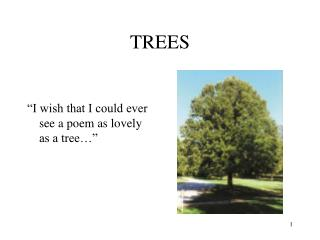DownloadDownload PresentationTREES

# TREES

Télécharger la présentation## TREES

- - - - - - - - - - - - - - - - - - - - - - - - - - - E N D - - - - - - - - - - - - - - - - - - - - - - - - - - -
##### Presentation Transcript

1. TREES “I wish that I could ever see a poem as lovely as a tree…”

2. TREES hierarchical structures parent-child relationship

3. A B C D E F TREES • The parent-child relationship exists between nodes. • A is the parent node of B and C • B,C are children of A • B, C are called siblings as they have the same parent

4. A B C D E F TREES • Each node in a tree has at most one parent. • Exactly one node - called the root of the tree- has no parent. • Node A is the root of this figure. • A node with no children is called a leaf.

5. A B C D E F SUBTREES •A generalization of the parent-child relationship is the ancestor-descendant relationship. • A is an ancestor of D, and thus D is a descendant of A. • A subtree in a tree is any node in the tree together with all its descendants.

6. General Trees • A general treeT is a set of one or more nodes such that T is partitioned into disjoint subsets: • A single node r, the root • Sets that are general trees, called subtrees of r.

7. Binary Trees • A binary tree is a set T of nodes such that either: • T is empty, or • T is partitioned into three disjoint subsets: • A single node r, the root • Two possibly empty sets that are binary trees, called left and right subtrees of r.

8. Binary Trees • T is a binary tree if either: • T has no nodes, or • T is of the form r TL TR where r is a node and TL and TR are both binary trees

9. Binary Search Trees A binary search tree (BST) is a binary tree that is, in a sense, sorted according to the values in its nodes.

10. Binary Search Trees • For each node n, a BST satisfies the following three properties: • n’s value is greater than all values in its left subtree TL • n’s value is less than all values in its right subtree TR • Both TL and TR are BST’s

11. 40 25 50 20 30 A BST of Integers

12. John Ben Ron Al Ellen Nell Sam A BST of Names

13. Shape Attributes of Trees • As we insert, delete, and search nodes in the tree, the “shape” of the tree affects the efficiency of the process. • The more “bushy” or uniform the tree, the more efficient the job becomes.

14. Shape Attributes of Trees • Among the attributes of trees that describe its shape include: • full • complete • balanced

15. A Full Binary Tree • A full binary tree has a "full" complement of nodes at every level of the tree. A full binary tree with 7 nodes A full binary tree of height h has 2h+1-1 nodes

16. A Complete Binary Tree • A complete binary tree has a full complement of nodes at each level above the leaf level… • …and the nodes at the highest leaf level must be filled in from the left. • A full binary tree is necessarily complete.

17. Some Complete Binary Trees

18. A Balanced Binary Tree • A binary tree is balanced if no two nodes of the following types have more than a single height distance between them: • leaf node • a node with only one child • Each full binary tree and each complete binary tree is necessarily balanced.

19. Balanced Binary Trees

20. Binary Tree Traversals • Once the tree is has been populated with data, processing(e.g., displaying data) each node is frequently a common task. • The process of referencing each node of the tree is called “traversing”. • Because of the recursive nature of the definition of binary trees, binary tree traversals are simplified by the use of recursion.

21. Binary Tree Traversals • The three(3) conventional binary tree traversals are: • preorder • inorder • postorder

22. 1 2 5 3 4 6 Preorder Traversal Traverse(T) { if T is not empty { print the data in the root of T Traverse(the left subtree of T, TL ) Traverse(the right subtree of T, TR ) } }

23. 4 2 6 1 3 5 Inorder Traversal Traverse(T) { if T is not empty { Traverse(the left subtree of T, TL ) print the data in the root of T Traverse(the right subtree of T, TR ) } }

24. 6 3 5 1 2 4 Postorder Traversal Traverse( T ) { if T is not empty { Traverse(the left subtree of T, TL ) Traverse(the right subtree of T, TR ) print the data in the root of T } }